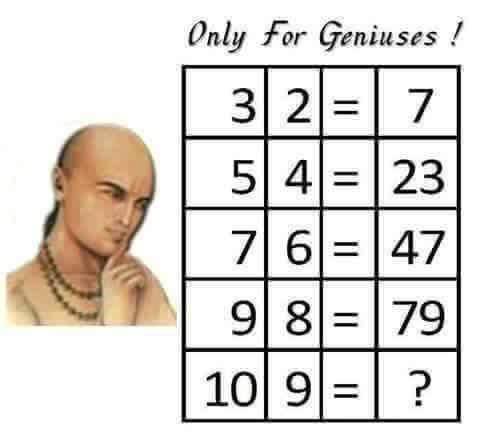# July 2016 Brain Teaser Solution

Q: In the town of Mathville, Anne is 5 years old.
The composition of the families in Mathville are as follows:
2% include five or more children
7% include four children
14% include three children
31% include two children
16% include one child
30% do not include any children

What are the chances that Anne lives with two brothers and no sisters?
(Consider all children living in any given house as siblings)

First we have to assume that there is a 50-50 chance for each child’s gender (although studies have shown if you already have 2 boys, you are likelier to have another son rather than a daughter).
We also have to assume that 5 is the number of children of the very large families.

Since Anne is a child, she cannot possibly live in a household with no children.
There is a (3×14)/(0 x 30 + 1 x 16 + 2 x 31 + 3 x 14 + 4 x 7 + 5 x 2)
or 26.582% chance that she lives with two other children.   Now we have to figure out the probability that both of the other children are boys.

There is a 50% chance that each of these other children is a boy, so there is a 25% chance that they are both boys (the combinations for the two other children are: BB, BG, GB and GG),   Therefore, the probability that Anne lives with two brothers is 25% of 26.582%, or about 6.6%.

# June 2016 Brain Teaser Solution

Q: The following equations are incorrect.  Fix them using these rules.
No other numbers can be written.
No inequality symbols can be used like a “greater than” sign, a “less than” sign or “not equal to” sign.1 1 1 =6
2 2 2 =6
3 3 3 =6
4 4 4 =6
5 5 5 =6
6 6 6 =6
7 7 7 =6
8 8 8 =6
9 9 9 =6
0 0 0 =6There can be multiple answers for one equation.

Hint: you can use ! and square roots
A:
(1+1+1)! = 3! = 3 x 2 x 1 = 6
2 + 2 + 2 = 6
3 x 3 – 3 = 6
4 + 4/sqrt(4) = 6
5 + 5/5 = 6
6 + 6 – 6 = 6
7 – 7/7 = 6
8 – sqrt(sqrt(8 + 8) = 2
9/sqrt(9)  + sqrt(9) = 6
(0! + 0! +0!)! = 6

# May 2016 Brain Teaser Solution3 x 2 + 1 = 7
5 x 4 + 3 = 23
7 x 6 + 5 = 47
9 x 8 + 7 = 79
10 x 9 + 8 = 98

# April 2016 Brain Teaser Solution

Q: Removing one from eleven makes it ten, removing one from nine makes it ten.
How is that?

A. Roman numerals
Eleven is XI, Nine is IX whereas ten is just X!

# February 2016 Brain Teaser Solution

Some people think January 1, 2000 was the first day of the 21st century. Others think it was January 1, 2001. But everyone should agree that January 1, 2002 was the first “sum-day” of the new century- when you write out the date in numbers, 01/01/02, and 1+1=2. A sum-day is a date in which the day and month add up to the last two digits of the year. With that in mind:
A) What is the last sum-day of the 21st century?
B) How many sum-days are there in the 21st century?